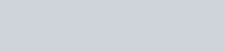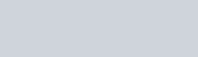Solve the following systems of equations graphically:
Question:

Solve the following systems of equations graphically:

$2 x-3 y+13=0$

$3 x-2 y+12=0$

Solution:

The given equations are:

$2 x-3 y+13=0$  $.(i)$

$3 x-2 y+12=0$ ..(ii)

Putting $x=0$ in equation $(i)$, we get:

$\Rightarrow 2 \times 0-3 y=-13$

$\Rightarrow y=13 / 3$

$x=0, \quad y=13 / 3$

Use the following table to draw the graph.Draw the graph by plotting the two points $A(0,13 / 2)$ and $B(-13 / 2,0)$ from table.Graph of the equation…. (ii):

$3 x-2 y=-12$ (ii)

Putting $x=0$ in equation $($ ii $)$ we get:

$\Rightarrow 3 \times 0-2 y=-12$

$\Rightarrow y=6$

$x=0, \quad y=6$

Putting $y=0$ in equation $(i i)$, we get:

$\Rightarrow 3 x-2 \times 0=-12$

$\Rightarrow x=-4$

$x=-4, \quad y=0$

Use the following table to draw the graph.Draw the graph by plotting the two points $C(0,6)$ and $D(-4,0)$ from table.

The two lines intersect at points $P(-2,3)$.

Hence, $x=-2$ and $y=3$ is the solution.